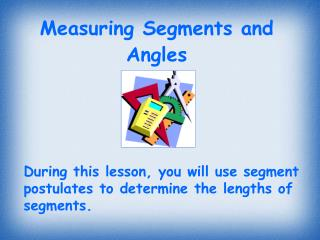DownloadDownload PresentationMeasuring Segments and Angles

# Measuring Segments and Angles

Télécharger la présentation## Measuring Segments and Angles

- - - - - - - - - - - - - - - - - - - - - - - - - - - E N D - - - - - - - - - - - - - - - - - - - - - - - - - - -
##### Presentation Transcript

1. Measuring Segments and Angles During this lesson, you will use segment postulates to determine the lengths of segments.

2. A B 3 0 -2 Segment Vocabulary distance and direction from 0 on a number line A coordinate is a point’s Ex. Point A is Point B is AB = _____units 2 units left of 0 3 units right of 0 5 Read: “the measure of line segment AB = 5”

3. A B 3 0 -2 is the absolute value of their difference. Ex. AB = │-2-3│= _____ AB = │-2-3│= _____

4. Use AB  CD (bar on top) when referring to the actual segments. Congruent () segments are segments with the same length. Notation for congruent segments: Read: “segment AB is congruent to segment CD” Use AB = CD (no bar on top) when referring to the lengths (or #’s). Read: “ measure of segment AB is equal to measure of segment CD”

5. 2cm. 2cm. AB  CD A C A B D B C D Actual segments Lengths (or numbers) AB = CD mAB = mCD

6. Q R S QR  RS m QR = m RS R is the midpoint of QS therefore: A midpoint is a point that __________________________________. divides a segment into two  segments. QR = RS

7. C B A POSTULATE Segment Addition Postulate If three points A, B and C are collinear and B is between A and C, then AB + BC = AC

8. Ex. #1 If DT = 60, find the value of x. Then find DS and ST. 3x - 12 2x - 8 T D S DS + ST = DT Segment Addition Postulate Substitute 2x - 8 + 3x - 12 = 60 Solve for x 5x - 20 = 60 5x = 80 DS= 2x - 8 x = 16 ST= 3x - 12 DS = 2(16) - 8 ST = 3(16) - 12 ST = 36 DS = 24

9. A B C D E F G -6 -2 0 5 3 8 7 EX. #2 Comparing Segment Lengths Compare AD and BF. 9 9 So, AD = BF AD = BF = Compare BD and EG. 5 3 BD = So, BD > EG EG =

10. EX. #3 Using the Midpoint 8x - 36 5x + 9 T R M M is the midpoint of RT. Find RM, MT and RT. RM = MT Def. of midpt. 5x + 9 = 8x - 36 Substitute RM = 5x + 9 45 = 3x Solve for x RM = 5(15) + 9 15 = x RM = 84 = MT RT = 84 + 84 = 168

11. Final Checks for Understanding • What is a postulate? • Draw a sketch of three collinear points. Label them. Then write the Segment Addition Postulate for the points. • Use the diagram. How can you determine BD if you know BC and CD? C D A B

12. Homework Assignment: Pages 29-33, text: #1-15, 29-32, 42-46, 71-72